# MCQs on Electric Circuits

##### Page 48 of 63. Go to page 1 2 3 4 5 6 7 8 9 10 11 12 13 14 15 16 17 18 19 20 21 22 23 24 25 26 27 28 29 30 31 32 33 34 35 36 37 38 39 40 41 42 43 44 45 46 47 48 49 50 51 52 53 54 55 56 57 58 59 60 61 62 63
01․ The rms value of sine wave is 100 A. Its peak value is
70.7 A.
141.4 A.
150 A.
282.8 A.

For sinusoidal alternating current the peak factor or amplitude factor can be expressed the ratio of maximum or peak value and rms value of alternating current. So the peak value = rms value of alternating current × peak factor of alternating current = 100 × 1.414 = 141.4 A.

02․ An alternating voltage = 140 sinθ is supplied to a device which offers a resistance of 20 Ω in forward direction of current in reverse direction. Calculate the form factor.
0.57.
0.318.
1.414.
1.11.

For alternating sinusoidal current form factor is defined as the ratio of rms value and average value of alternating current. Now the rms value of sinusoidal alternating current = 0.07 × maximum value of alternating current. Average value of sinusoidal alternating current = 0.637 × maximum value of alternating current.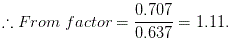03․ A network contains linear resistors and ideal voltage sources. If values of all the resistors are doubled, then voltage across each resistor is
halved.
doubled.
increase by two times.
remain same.

V is a constant. If R is doubled then, current will become half. So voltage across each resistor remains same.

04․ The voltage across the 1kΩ resistor between the nodes A and B of the network shown in the given figure is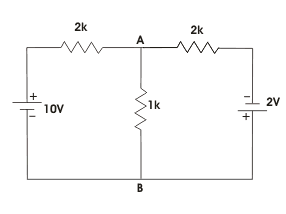2 V.
1 V.
-1 V.
-2 V.

Applying KCL at node A, VA = 2V.

05․ An electrical circuit with 10 branches and 7 nodes will have
10 loop equations.
7 loop equations.
3 loop equations.
4 loop equations.

Number of loop equations = branches - nodes + 1 = b - n + 1 = 10 - 7 + 1 = 4 loop equations.

06․ In given figure, the value of resistance R in Ω is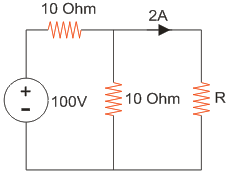10.
20.
30.
40.

The given circuit can be simplified by replacing voltage source by equivalent current source as below,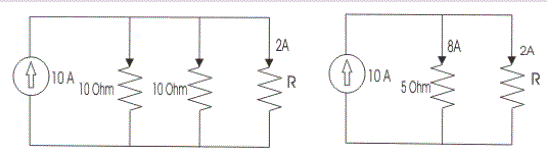The current through 5 Ω resistor is 8 A hence voltage across it is 8 × 5 = 40 V and this is the voltage across unknown resistor R. As current through R is 2 A.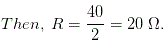07․ In given figure, the value of resistance R in Ω is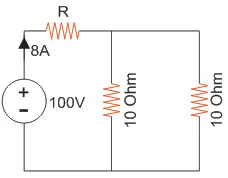2.5 Ω.
5 Ω.
7.5 Ω.
10 Ω.

The given circuit can be simplified by replacing voltage source by equivalent current source as below,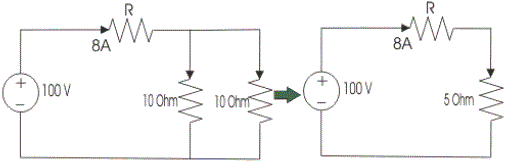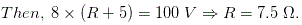08․ A 35 V source is connected to a series circuit of 600 Ω and R as shown. If a voltmeter of internal resistance 1.2 kΩ is connected across 600 Ω resistor, it reads 5 V. The value of R is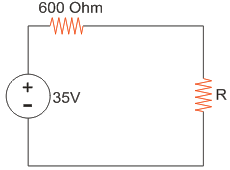2.4 kΩ.
1.2 kΩ.
3.6 kΩ.
7.2 kΩ.

As the voltmeter of internal resistance 1.2 kΩ is connected across the 600 Ω resistor and it gives 5 V reading.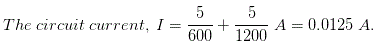As supply voltage is 35 V, the voltage across resistor R is 35 - 5 = 30 V.

09․ Find the current through 5 Ohm resistor from A to B.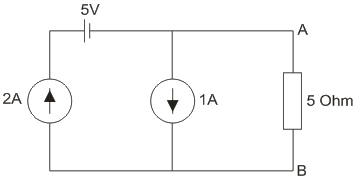2A.
1A.
-2A.
-1A.

Applying Superposition theorem, current due to 5V source is zero; current due to 2A source is 2A from A to B; current due to 1A source is 1A from B to A. So total current from A to B due to all the sources is 1A.

10․ In the network shown, what is the current I in the direction shown?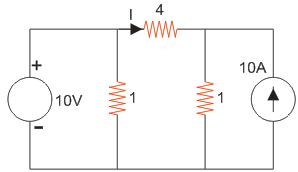1 A.
-1 A.
0
2 A.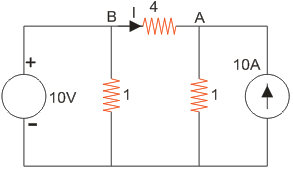VB = 10V; Applying KCL at A, VA = 10V; So no current flows between them.

<<<4647484950>>>